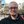davedarko wrote 09/27/2016 at 19:28 • 5 min read • Like

This is my write up of how I made the cheap Chinese knockoff xbox chatpad to work for me.

After watching one of Ben Hecks classics - "Build a Retro Computer: BASIC 80's Pocket Computer"[link] I bought the cheapest on eBay I was able to find (as always), but quickly found out that they aren't the same and as easily reprogrammable. A bit frustrated I left this in a drawer for a while, until recently, where I was looking for a good portable keyboard for my #Portable Raspberry PI Zero project. So my solution was to add an Arduino Pro Micro with an Atmega32u4 on it, that has native USB and is also to find on a Teensy 2.0. I had to remove the IC blob on the Keyboard PCB first, to make sure it wouldn't be powered by the row scanning and crosstalk, then hooked up the Arduino to the test points. I also worked out some modifiers but got lazy with the green ones.``` #include "Keyboard.h"
// TP1, TP20, TP21, TP22, TP23, TP24, TP25
int rows = {3, 6, 7, 8, 5, 4, 2};
// TP6, TP7, TP8, TP9, TP10, TP11, TP12
int cols = {9, 19, 18, 15, 14, 16, 10};
int BACKSPACE = -1;
int LEFT = -2;
int RIGHT = -3;
int GREEN = -4;
int RED = -5;
int CHAT = -6;
int ENTER = -7;
int SHIFT = -8;
int NORMAL = -9;
boolean is_shift=false, is_green=false, is_red=false, is_normal=true;
char characters  =
{
// 6    7    8   9    10   11   12
{BACKSPACE, 'k', 'i', 'o', ' ', ' ', 'l'}, // TP1
{'7', '1', '2', '3', '4', '5', '6'}, // TP20
{'u', 'q', 'w', 'e', 'r', 't', 'y'}, // TP21
{'j', 'a', 's', 'd', 'f', 'g', 'h'}, // TP22
{'n', SHIFT, 'z', 'x', 'c', 'v', 'b'}, // TP23
{RIGHT, GREEN, CHAT, LEFT, ' ', '.', 'm'}, // TP24
{RED, '8', '9', '0', 'p', ENTER, ','} // TP25
};
char shift_characters  =
{
// 6    7    8   9    10   11   12
{BACKSPACE, 'K', 'I', 'O', ' ', ' ', 'L'}, // TP1
{'7', '1', '2', '3', '4', '5', '6'}, // TP20
{'U', 'Q', 'W', 'E', 'R', 'T', 'Y'}, // TP21
{'J', 'A', 'S', 'D', 'F', 'G', 'H'}, // TP22
{'N', SHIFT, 'Z', 'X', 'C', 'V', 'B'}, // TP23
{RIGHT, GREEN, CHAT, LEFT, ' ', '.', 'M'}, // TP24
{RED, '8', '9', '0', 'P', ENTER, ','} // TP25
};
char red_characters  =
{
// 6    7    8   9    10   11   12
{BACKSPACE, 'k', 'i', 'ö', ' ', ' ', 'ø'}, // TP1
{'7', '1', '2', '3', '4', '5', '6'}, // TP20
{'u', 'q', 'w', 'e', 'r', 't', 'y'}, // TP21
{'"', 'ä', 'ß', 'd', '£', '¥', '|'}, // TP22
{'n', SHIFT, 'z', 'x', 'ç', '_', '+'}, // TP23
{RIGHT, GREEN, CHAT, LEFT, ' ', '¿', 'µ'}, // TP24
{RED, '8', '9', '0', '=', ENTER, ';'} // TP25
};
char green_characters  =
{
// 6    7    8   9    10   11   12
{BACKSPACE, '[', '*', '(', ' ', ' ', 'l'}, // TP1
{'7', '1', '2', '3', '4', '5', '6'}, // TP20
{'&', '!', '@', '€', '#', '%', '^'}, // TP21
{'\'', '~', 'š', '{', '}', '¨', '/'}, // TP22
{'<', SHIFT, '`', '«', '»', '-', '|'}, // TP23
{RIGHT, GREEN, CHAT, LEFT, ' ', '?', '>'}, // TP24
{RED, '8', '9', '0', ')', ENTER, ':'} // TP25
};
void setup()
{
// put your setup code here, to run once:
for (int i = 0; i < 7; i++)
{
pinMode(rows[i], OUTPUT);
}
for (int i = 0; i < 7; i++)
{
pinMode(cols[i], INPUT_PULLUP);
}
Keyboard.begin();
}
void loop()
{
// put your main code here, to run repeatedly:
for (int i = 0; i < 7; i++)
{
digitalWrite(rows[i], LOW);
for (int j = 0; j < 7; j++)
{
if (pinstate == LOW)
{
if (characters[i][j] == ENTER)
{
Keyboard.press(KEY_RETURN);
Keyboard.release(KEY_RETURN);
}
else if (characters[i][j] == BACKSPACE)
{
Keyboard.press(KEY_BACKSPACE);
Keyboard.release(KEY_BACKSPACE);
}
else if (characters[i][j] == RIGHT)
{
Keyboard.press(KEY_RIGHT_ARROW);
Keyboard.release(KEY_RIGHT_ARROW);
}
else if (characters[i][j] == LEFT)
{
Keyboard.press(KEY_LEFT_ARROW);
Keyboard.release(KEY_LEFT_ARROW);
}
if (characters[i][j] == SHIFT)
{
set_states(SHIFT);
}
else if (characters[i][j] == GREEN)
{
set_states(GREEN);
}
else if (characters[i][j] == RED)
{
set_states(RED);
}
if (characters[i][j] > 0)
{
if (is_shift) Keyboard.write(shift_characters[i][j]);
if (is_green) Keyboard.write(green_characters[i][j]);
if (is_red) Keyboard.write(red_characters[i][j]);
if (is_normal) Keyboard.write(characters[i][j]);
set_states(NORMAL);
}
delay(250);
}
}
digitalWrite(rows[i], HIGH);
}
}
//
void set_states(int state)
{
is_shift = (state==SHIFT);
is_green = (state==GREEN);
is_red = (state==RED);
is_normal = !(is_shift||is_green||is_green);
//
//  Keyboard.print(is_shift?"1":"0");
//  Keyboard.print(is_green?"1":"0");
//  Keyboard.print(is_red?"1":"0");
//  Keyboard.print(is_normal?"1":"0");
}
```

## Discussions